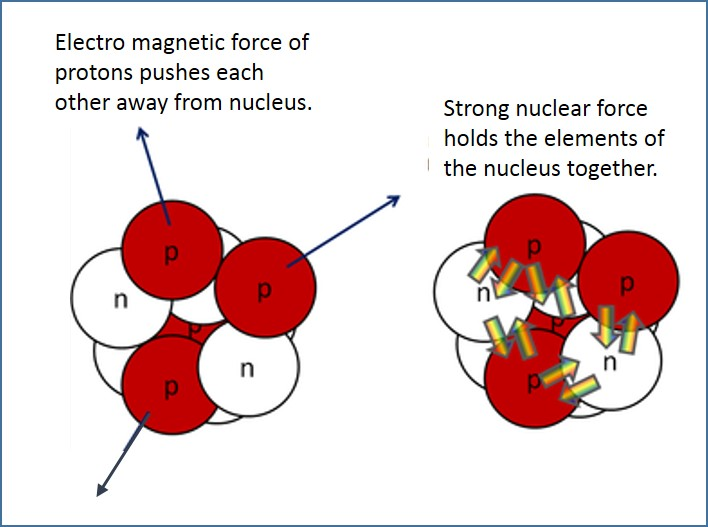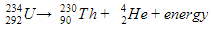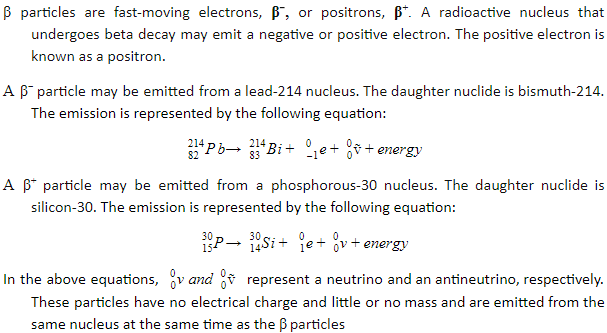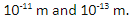# Stable & Unstable Nuclei

## Introduction

An atom contains an equal number of positively charged protons and negatively charged electrons and their charges balance. However, the nucleus contains positively charged protons, which are closely packed together in a very small volume. According to the laws of physics, the protons exert strong repulsive forces on each other. This implies that there must be an additional attractive force similar in size to the electrostatic repulsion that holds the nucleus together. In this article, forces present in the nucleus, stable and unstable atoms, and particles emitted in the decay of the unstable atoms, are explained.

A. Strong Nuclear Force

The attractive force, similar in size to the electrostatic repulsion, which holds the nucleus together, is called the strong nuclear force. The electromagnetic force and strong nuclear force present in the nucleus are illustrated in Figure 1.Figure 1: Forces in the Nucleus

A stable nucleus is a nucleus that has enough binding energy to hold the elements of the nucleus together permanently. In unstable nuclei, the strong nuclear forces do not generate enough binding energy to hold the elements of the nucleus together permanently. Hadrons (Mesons & Baryons) experience the strong nuclear force but Leptons do not. The strong nuclear force acts over a very short range. It can be both attractive and repulsive.

B. Decay of Unstable Nuclei

The unstable nuclei, in order to become stable nuclei, emit particles and/or electromagnetic radiation. These types of nuclei are said to be radioactive, and the emission is called radioactivity. The three type of emission that originate from the nucleus are:

• Alpha particles (α)
• Beta particles (β)
• Gamma particles (γ).

C. Alpha Particles (α)

An alpha particle is identical to the nucleus of a helium atom. It contains two protons and two neutrons. So, it carries a charge of +2e. An alpha particle is written as 24He. When a nucleus of an atom emits an alpha particle, it is said to undergo alpha decay. The nucleus loses two protons and two neutrons in this emission. Each element has a particular number of protons, and therefore α decay causes one element to change into another. This process is also called transmutation. The original nuclide is called the parent nuclide, and the new one is the daughter nuclide.

For example, uranium-234 may emit α particles. The daughter nuclide in this reaction is thorium-230. The emission is represented by the following equation:D. Beta Particles (β)E. Gamma Particles (γ)

Alpha and beta particles are emitted by unstable nuclei, which have excess energy. The emission of these particles results in changes in the ratio of protons to neutrons, but the nuclei may still have excess energy. The nucleus may return to its unexcited or stable state by emitting energy in the form of γ particles. γ−radiation is part of the electromagnetic spectrum with wavelengths betweenFor example, when uranium-238 decays by emitting an α particle, the resulting nucleus of thorium-234 contains excess energy and emits a photon of γ−radiation to return to the ground level. The emission process is represented by the following equation. Thorium in an excited state is represented byF. Neutrino and Antineutrino Particles

In β-decay, neutrino and antineutrino particles are emitted. These particles have no electrical charge and little or no mass and are emitted from the same nucleus at the same time as the β particles.

G. Summary

• The attractive force, similar in size to the electrostatic repulsion, which holds the elements of the nucleus together, is called as the strong nuclear force.
• An unstable nucleus, in order to become a stable nucleus, emits α, β or γ particles and/or electromagnetic radiation.
• A radioactive nucleus that undergoes alpha decay emits alpha particles. It contains two protons and two neutrons.
• A radioactive nucleus that undergoes beta decay may emit a negative or positive electron.
• The nucleus may return to its unexcited or stable state by emitting energy in the form of γ particles.